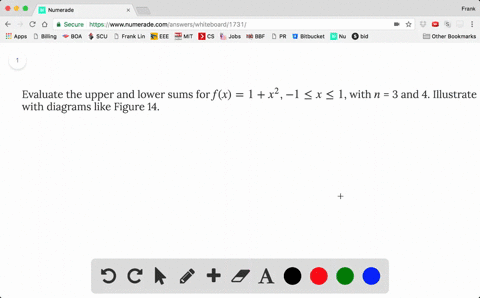8.65

## Discussion

You must be signed in to discuss.

## Video Transcript

Okay, So the value is operas on the lower self Well, here I'Ll explain the concept and I'll let you work out the details. So basically previously with know howto devalue our estimates the area by using the right and point out that in their temples or middle part here are upper And the lower sums means that after we take the rectangle itself picking certain en pointe Upper Ming's we always picks a maximum of the function that interval and lower means we always pick Ah minimum at on that Rick Congo is the height of their on that intervals the height of the rectangle So here are use and it was for to explain So our inter voice from zero to pie So we have zero here, here, here. So we have pi over four Hi over too Hi over three I'm so sorry Enough high over three Should be three pile were for and see what happened So upper means For each interval we pick the largest possible value ofthe f at that point So we know what is the function of science? Looks like by the way from zero to pie it goes from zero to one at Pi over too. And they go Bacs to part Coco Best zero from pi over to the party. So this is like aah! Not F issues. Is that a graph of function science? So here, if you want to use it if you want to come to the opera some forage Interval We have the Pixar largest one off f us our height of the rogue tango. So for the first interval, with against science increasing so largest one sign pi over four. So here we pick f off pi over four and this one against science increasing on this interval. So the largest ones therefore pie over too. How about this one? Now this efforts decrease your science started cuisine so the larger slice also have pie over too. How about this one again? Efforts decreasing, Czar. Large largest ones. The left end point f off three pi over four Have a lower some lower summer each interval. Wrong pick the smallest one from zero to pile For efforts decreasing us. Sorry, f is increasing. So So the left hand points the smallest one here f it's increasing. So the left end points the smallest one here f decreasing. Remember science. Start decreasing after pie over, too. So it's a the smallest ones. Serve right in point three pi over four and again this one's decreasing a funk, this intervals decreasing, so the smallest ones are right on point. So what is upper someone? Lower, son? That's the sum of the areas of the wreck chemical. We know that the base of each rock tengo is pi over four. So opera some is hi over for times F off pi over four Vaziri at thes number together plus f pie over too. Plus another F Opie over two plus f off. No, let's use another page May be easier. So the uppers or I guess, Ah, sorry. Is there some problem here? I leave it to you the royal detail. But basically the upper some the upper some is pi over four times sum of all these things and the lower some is pi over four times sum of all these things. Oh, because you know, you add them together Times pi over four. That's opera song and the lower some again you add them together times pi over four And remember what its f f It's dysfunction. So So that's your upper for some and lower some, and you can apply similar concept to other possible on values.